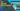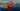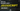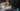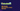# What are Loops in C Sharp and How to Use It?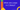What are Loops? After learning Interfaces in C# in our previous C Sharp Article, let’s learn Loops in C Sharp, normally when we want to execute a statement once we mention it once in our program and execute the program. But what if you need to repeat a statement multiple times. Sometimes you know how many times you need to repeat the statement and sometimes you don’t. How can we overcome this problem? The answer by Microsoft is a loop. There are several types of loops that can help you solve such problems and all the similar types of problems.

## Loops in C Sharp

Loops in C Sharp allow us to repeat a single statement or a block of statements multiple times. When you know how many times the loop should repeat the statement, you mention it in the loop. The would repeat itself exactly how many times you want it to. Sometimes you dont know how many times the loop should repeat itself, in this case we need to give loop a condition to stop. According to a condition the loop will be stopped.

In case we don’t mention when the loop should stop itself, it will keep on running and never stop. There is a loop that comes with the condition to stop. That loop is called the foreach loop. I will be explaining the structure of the loop and also explain all the loops for you with examples.

## Types of Loops in C Sharp

1. For Loop
2. While Loop
3. Do While Loop
4. Foreach Loop

## For Loop

We use ‘For loop’ when we know how many times we need to repeat the execution. For example, we want to print the number from 1 to 100 times. The first way of doing is writing the statement a hundred times and the second way of doing is using the ‘For Loop’.

Look at the structure of ‘For Loop’ below. You can see the signature of the ‘For Loop’. In signature we have 3 parts, the first part is the initialization of variable ‘i’, the second part is the length of ‘i’, and the third and final part is the increment or decrement of ‘i’. Each time when the loop is repeated, the code inside { } is executed. Don’t worry if you did not understand it, I will explain it to you.

``````for (int i = 0; i < length; i++)
{
}``````

Remember we wanted to print the number from 1 to 100 times. The variable ‘i’ will hold the value of ‘i’. In the first part, we will mention what should be the initial value of ‘i’. We will set it as 1 because we want to start the count from 1. In the next part, we will mention how many times we want to repeat the statement, this is why we will set the length as less than or equal to 100. The third part is saying we will increase the value of ‘i’ by 1 every time when the statement is executed.

``````using System;
namespace Loops
{
class Program
{
static void Main(string[] args)
{
for (int i = 1; i <= 100; i++)
{
Console.WriteLine(i);
}
}
}
}
``````

## Explanation

You can see in the program above, the statement that we want to repeat is mentioned inside the For Loop block. In the signature of the loop, we have already mentioned we want to start the count from 1 and want it to be repeated till the value of ‘i’ reaches 100.

Note – We can not write the length as i = 100, we will have to write it as ‘i <=100’. Also, note that we use the same loop to print the number in reverse order as well. In case we want to print from 100 to 1. Here is how we can do it.

``````using System;
namespace Loops
{
class Program
{
static void Main(string[] args)
{
for (int i = 100; i >= 1; i--)
{
Console.WriteLine(i);
}
}
}
}
``````

In the above program, you can see we are starting the count from 100 and set the length as >= 1, the incrementer becomes decrementer. Every time when the loop runs, the value of ‘i’ decreases by 1.

I hope the article was helpful. I will be writing more articles explaining While Loop and Do While loop. Till then stay tuned.

Tags

Search by